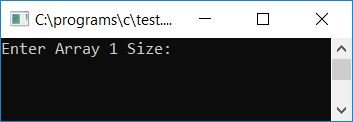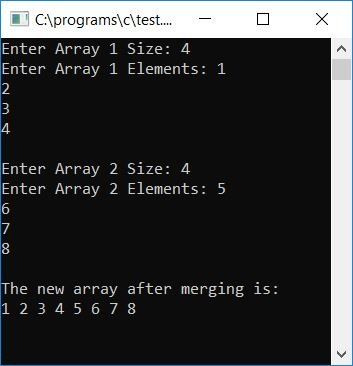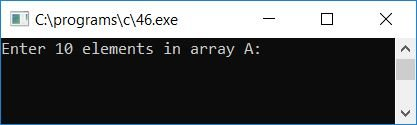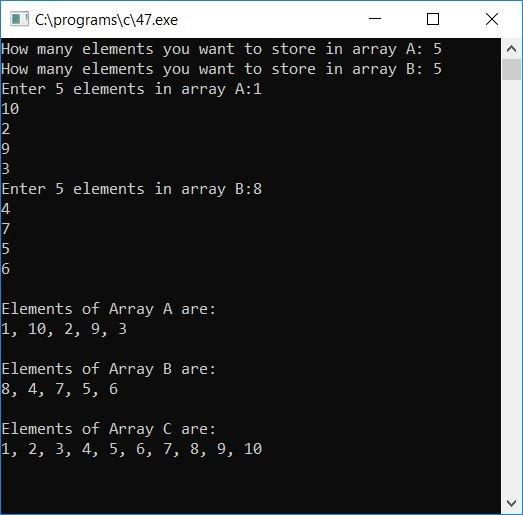# C Program to Merge Two Arrays

In this article, we will learn how to merge any two arrays to form a third array that contains all the elements of the two given arrays. We will also learn about merging arrays in ascending and descending order.

## Merge Two Arrays in C

To merge any two arrays in C programming, start adding each and every element of the first array to the third array (the target array). Then start appending each and every element of the second array to the third array (the target array), as shown in the program given below.

The following C program asks the user to enter "array 1" size and its elements, then "array 2" size and its elements to merge and form the new array (the target array or third array), then display the result of the merged array. Here we have displayed the merged array directly. You can also sort the two arrays before merging. So to learn about sorting, here is a list of three techniques to sort any array:

Now let's take a look at the program for merging two given arrays. The question is: write a program in C to merge any two arrays entered by the user at run-time. The answer to this question is:

```#include<stdio.h>
#include<conio.h>
int main()
{
int arr1, arr2, size1, size2, i, k, merge;
printf("Enter Array 1 Size: ");
scanf("%d", &size1);
printf("Enter Array 1 Elements: ");
for(i=0; i<size1; i++)
{
scanf("%d", &arr1[i]);
merge[i] = arr1[i];
}
k = i;
printf("\nEnter Array 2 Size: ");
scanf("%d", &size2);
printf("Enter Array 2 Elements: ");
for(i=0; i<size2; i++)
{
scanf("%d", &arr2[i]);
merge[k] = arr2[i];
k++;
}
printf("\nThe new array after merging is:\n");
for(i=0; i<k; i++)
printf("%d ", merge[i]);
getch();
return 0;
}```

As the above program was written in the Code::Blocks IDE, therefore, after a successful build and run, you will get the following output on your output screen:Now supply the size and elements of the first array, and then the size and elements of the second array. Press the ENTER key to see the merged array as output:#### Program Explained

• Receive the size of the first array from the user at program runtime; say "4".
• Then receive 4 elements for the first array.
• After receiving the first array's elements using the for loop, set up each element in the "merge" array.
• That is, the first, second, third, and fourth elements of the first array are initialized in the merging array at merge, merge, merge, and merge.
• Following the inputs of all four elements for the first array
• Initialize the value of variable i to any other variable, say k. Now k holds the value 4 (in this case).
• Receive the size of the second array, say 4.
• Then receive 4 elements for the second array.
• Set up each element in the "merge" array after receiving the second array elements using the for loop in a similar way as done while receiving the first array. This time, start the merge array's index from where the last indexing ends, which is 4 (the starting value of k).
• That is, the first, second, third, and fourth elements of the second arrays are set up in the merging array at merge, merge, merge, and merge.
• Create a loop that goes from 0 to the value that the variable k holds, in this case, 0 to 7.
• and print the merged array inside the loop.

## Merge Two Arrays in Ascending Order

The question is: write a program in C to read values from two arrays, A and B, and merge elements of both arrays into a third array, C, in ascending order. Finally, print the entire array. The answer to this question is given below:

```#include<stdio.h>
#include<conio.h>
int main()
{
int a, b, c, i, j, limitC, temp;
printf("Enter 10 elements in array A:");
for(i=0; i<10; i++)
scanf("%d", &a[i]);
printf("Enter 10 elements in array B:");
for(i=0; i<10; i++)
scanf("%d", &b[i]);
printf("\nElements of Array A are:\n");
for(i=0; i<10; i++)
{
if(i==9)
printf("%d", a[i]);
else
printf("%d, ", a[i]);
}
printf("\n\nElements of Array B are:\n");
for(i=0; i<10; i++)
{
if(i==9)
printf("%d", b[i]);
else
printf("%d, ", b[i]);
}

// merging the two arrays
for(i=0; i<10; i++)
c[i] = a[i];
for(j=0; j<10; j++)
{
c[i] = b[j];
i++;
}

// sorting the merged array
for(j=0; j<19; j++)
{
for(i=0; i<19; i++)
{
if(c[i]>c[i+1])
{
temp = c[i];
c[i] = c[i+1];
c[i+1] = temp;
}
}
}
printf("\n\nElements of Array C are:\n");
for(i=0; i<20; i++)
{
if(i==19)
printf("%d", c[i]);
else
printf("%d, ", c[i]);
}
getch();
return 0;
}```

Here is the first snapshot of the sample run:Now supply any 10 array elements for array A and then any 10 array elements for array B, and press the ENTER key to see three arrays. The first two arrays are the given arrays, and the third array contains all of the elements from arrays A and B and is arranged in ascending order.Here is the second snapshot:The same concept is used to merge the two arrays in the above program, except that we have used some extra code to arrange the merged array in ascending order. To arrange each and every element in ascending order

• Make a for loop that goes from 0 to the size of the array.
• and inside the for loop, create another for loop with different loop variables starting from 0 to the size of the array.
• and inside the second for loop, compare each and every element of the array.
• If the first array element is greater than the second one
• then put the first to the second, and the second to the first. Simply swapping will be performed here.
• Do the same thing until all the elements are arranged in ascending order.

### Allow the user to define the array size

This program does the same job as the previous program, which was to merge two given arrays in ascending order. The only difference is that this program allows the user to specify the array's size as well as its elements:

```#include<stdio.h>
#include<conio.h>
int main()
{
int a, b, c, limitA, limitB, i, j, limitC, temp;
printf("How many elements you want to store in array A: ");
scanf("%d", &limitA);
printf("How many elements you want to store in array B: ");
scanf("%d", &limitB);
printf("Enter %d elements in array A:", limitA);
for(i=0; i<limitA; i++)
scanf("%d", &a[i]);
printf("Enter %d elements in array B:", limitB);
for(i=0; i<limitB; i++)
scanf("%d", &b[i]);
printf("\nElements of Array A are:\n");
for(i=0; i<limitA; i++)
{
if(i==(limitA-1))
printf("%d", a[i]);
else
printf("%d, ", a[i]);
}
printf("\n\nElements of Array B are:\n");
for(i=0; i<limitB; i++)
{
if(i==(limitB-1))
printf("%d", b[i]);
else
printf("%d, ", b[i]);
}
for(i=0; i<limitA; i++)
c[i] = a[i];
for(j=0; j<limitB; j++)
{
c[i] = b[j];
i++;
}
limitC = i;
for(j=0; j<(limitC-1); j++)
{
for(i=0; i<(limitC-1); i++)
{
if(c[i]>c[i+1])
{
temp = c[i];
c[i] = c[i+1];
c[i+1] = temp;
}
}
}
printf("\n\nElements of Array C are:\n");
for(i=0; i<limitC; i++)
{
if(i==(limitC-1))
printf("%d", c[i]);
else
printf("%d, ", c[i]);
}
getch();
return 0;
}```

Here is the final snapshot of the sample run:## Merge Two Arrays in Descending Order

Now let's create another program that will merge the two given arrays in descending order:

```#include<stdio.h>
#include<conio.h>
int main()
{
int a, b, c, i, j, limitC, temp;
printf("Enter 10 elements in array A:");
for(i=0; i<10; i++)
scanf("%d", &a[i]);
printf("Enter 10 elements in array B:");
for(i=0; i<10; i++)
scanf("%d", &b[i]);
printf("\nElements of Array A are:\n");
for(i=0; i<10; i++)
{
if(i==9)
printf("%d", a[i]);
else
printf("%d, ", a[i]);
}
printf("\n\nElements of Array B are:\n");
for(i=0; i<10; i++)
{
if(i==9)
printf("%d", b[i]);
else
printf("%d, ", b[i]);
}

// merging the two arrays
for(i=0; i<10; i++)
c[i] = a[i];
for(j=0; j<10; j++)
{
c[i] = b[j];
i++;
}

// sorting the merged array
for(j=0; j<19; j++)
{
for(i=0; i<19; i++)
{
if(c[i]<c[i+1])
{
temp = c[i];
c[i] = c[i+1];
c[i+1] = temp;
}
}
}
printf("\n\nElements of Array C are:\n");
for(i=0; i<20; i++)
{
if(i==19)
printf("%d", c[i]);
else
printf("%d, ", c[i]);
}
getch();
return 0;
}```

Below is the final snapshot of the sample run:Now let's modify the above program and allow the user to create the limit or size for both the arrays. The question is: write a program in C to read the size and values of two arrays and merge elements of both arrays into a third array in descending order. Finally, print the entire array. The program given below is the answer to this question:

```#include<stdio.h>
#include<conio.h>
int main()
{
int a, b, c, limitA, limitB, i, j, limitC, temp;
printf("How many elements you want to store in array A: ");
scanf("%d", &limitA);
printf("How many elements you want to store in array B: ");
scanf("%d", &limitB);
printf("Enter %d elements in array A:", limitA);
for(i=0; i<limitA; i++)
scanf("%d", &a[i]);
printf("Enter %d elements in array B:", limitB);
for(i=0; i<limitB; i++)
scanf("%d", &b[i]);
printf("\nElements of Array A are:\n");
for(i=0; i<limitA; i++)
{
if(i==(limitA-1))
printf("%d", a[i]);
else
printf("%d, ", a[i]);
}
printf("\n\nElements of Array B are:\n");
for(i=0; i<limitB; i++)
{
if(i==(limitB-1))
printf("%d", b[i]);
else
printf("%d, ", b[i]);
}
for(i=0; i<limitA; i++)
c[i] = a[i];
for(j=0; j<limitB; j++)
{
c[i] = b[j];
i++;
}
limitC = i;
for(j=0; j<(limitC-1); j++)
{
for(i=0; i<(limitC-1); i++)
{
if(c[i]<c[i+1])
{
temp = c[i];
c[i] = c[i+1];
c[i+1] = temp;
}
}
}
printf("\n\nElements of Array C are:\n");
for(i=0; i<limitC; i++)
{
if(i==(limitC-1))
printf("%d", c[i]);
else
printf("%d, ", c[i]);
}
getch();
return 0;
}```

This is the final snapshot of the sample run:### Same Program in Other Languages

C Quiz

« Previous Program Next Program »